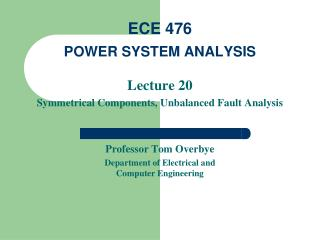Download PresentationECE 476 POWER SYSTEM ANALYSIS

# ECE 476 POWER SYSTEM ANALYSIS - PowerPoint PPT PresentationDownload Presentation## ECE 476 POWER SYSTEM ANALYSIS

- - - - - - - - - - - - - - - - - - - - - - - - - - - E N D - - - - - - - - - - - - - - - - - - - - - - - - - - -
##### Presentation Transcript

1. ECE 476POWER SYSTEM ANALYSIS Lecture 20 Symmetrical Components, Unbalanced Fault Analysis Professor Tom Overbye Department of Electrical andComputer Engineering

2. Announcements • Be reading Chapters 8 and 9 • HW 8 is 7.6, 7.13, 7.19, 7.28; due Nov 3 in class. • Start working on Design Project. Tentatively due Nov 17 in class • Second exam is on Nov 15 in class. Same format as first exam, except you can bring two note sheets (e.g., the one from the first exam and another)

3. Analysis of Unsymmetric Systems • Except for the balanced three-phase fault, faults result in an unbalanced system. • The most common types of faults are single line-ground (SLG) and line-line (LL). Other types are double line-ground (DLG), open conductor, and balanced three phase. • System is only unbalanced at point of fault! • The easiest method to analyze unbalanced system operation due to faults is through the use of symmetrical components

4. Symmetric Components • The key idea of symmetrical component analysis is to decompose the system into three sequence networks. The networks are then coupled only at the point of the unbalance (i.e., the fault) • The three sequence networks are known as the • positive sequence (this is the one we’ve been using) • negative sequence • zero sequence

5. Positive Sequence Sets • The positive sequence sets have three phase currents/voltages with equal magnitude, with phase b lagging phase a by 120°, and phase c lagging phase b by 120°. • We’ve been studying positive sequence sets Positive sequence sets have zero neutral current

6. Negative Sequence Sets • The negative sequence sets have three phase currents/voltages with equal magnitude, with phase b leading phase a by 120°, and phase c leading phase b by 120°. • Negative sequence sets are similar to positive sequence, except the phase order is reversed Negative sequence sets have zero neutral current

7. Zero Sequence Sets • Zero sequence sets have three values with equal magnitude and angle. • Zero sequence sets have neutral current

8. Sequence Set Representation • Any arbitrary set of three phasors, say Ia, Ib, Ic can be represented as a sum of the three sequence sets

9. Conversion from Sequence to Phase

10. Conversion Sequence to Phase

11. Conversion Phase to Sequence

12. Symmetrical Component Example 1

13. Symmetrical Component Example 2

14. Symmetrical Component Example 3

15. Use of Symmetrical Components • Consider the following wye-connected load:

16. Use of Symmetrical Components

17. Networks are Now Decoupled

18. Sequence diagrams for generators • Key point: generators only produce positive sequence voltages; therefore only the positive sequence has a voltage source During a fault Z+ Z  Xd”. The zero sequence impedance is usually substantially smaller. The value of Zn depends on whether the generator is grounded

19. Sequence diagrams for Transformers • The positive and negative sequence diagrams for transformers are similar to those for transmission lines. • The zero sequence network depends upon both how the transformer is grounded and its type of connection. The easiest to understand is a double grounded wye-wye

20. Transformer Sequence Diagrams

21. Unbalanced Fault Analysis • The first step in the analysis of unbalanced faults is to assemble the three sequence networks. For example, for the earlier single generator, single motor example let’s develop the sequence networks

22. Sequence Diagrams for Example Positive Sequence Network Negative Sequence Network

23. Sequence Diagrams for Example Zero Sequence Network

24. Create Thevenin Equivalents • To do further analysis we first need to calculate the thevenin equivalents as seen from the fault location. In this example the fault is at the terminal of the right machine so the thevenin equivalents are:

25. Single Line-to-Ground (SLG) Faults • Unbalanced faults unbalance the network, but only at the fault location. This causes a coupling of the sequence networks. How the sequence networks are coupled depends upon the fault type. We’ll derive these relationships for several common faults. • With a SLG fault only one phase has non-zero fault current -- we’ll assume it is phase A.

26. SLG Faults, cont’d

27. SLG Faults, cont’d

28. SLG Faults, cont’d With the sequence networks in series we can solve for the fault currents (assume Zf=0)